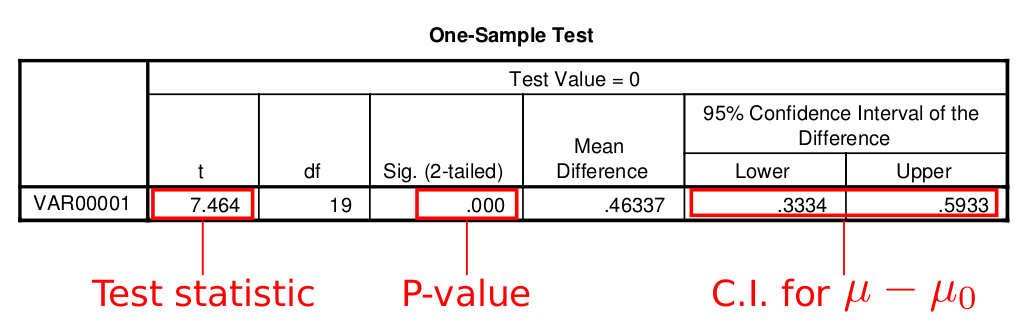Your browser doesn't support the features required by impress.js, so you are presented with a simplified version of this presentation.

For the best experience please use the latest Chrome, Safari or Firefox browser.

# Confidence Interval

• Example: estimate population mean $\mu$
• The sample mean $\bar{X}$, a single number, is usually not enough nor reliable.
• Instead, we use an interval of the form $$estimate \pm margin\ of\ error$$ to "capture" the true mean.

# C.I. for $\mu$

• If we know the population standard deviation $\sigma$, then the confidence interval for $\mu$ is $$\left(\bar{X}-z^*\frac{\sigma}{\sqrt{n}}, \bar{X}+z^*\frac{\sigma}{\sqrt{n}}\right)$$
• The bottom of the t-table gives the value of $z^*$ corresponding to different confidence level $C$
• $C=90\%\Rightarrow z^*=1.645$
• $C=95\%\Rightarrow z^*=1.960$
• $C=99\%\Rightarrow z^*=2.576$

# Hypothesis Testing

• Draw conclusion on a statement about the population
• Steps
• State null hypothesis and alternative hypothesis
• Calculate test statistic
• Find P-value
• Make conclusion

# State Hypotheses

• Want to test whether the populatoin mean $\mu$ is greater than $\mu_0$ or not $$H_0:\mu=\mu_0\quad H_a:\mu>\mu_0$$
• Want to test whether the populatoin mean $\mu$ is less than $\mu_0$ or not $$H_0:\mu=\mu_0\quad H_a:\mu<\mu_0$$
• Want to test whether the populatoin mean $\mu$ is equal to $\mu_0$ or not $$H_0:\mu=\mu_0\quad H_a:\mu\neq\mu_0$$

# Test Statistic

• If population standard deviation $\sigma$ is given, we calculate the z-score for $\bar{X}$ $$z=\frac{\bar{X}-\mu}{\sigma/\sqrt{n}}$$

# P-value

• $H_a:\mu>\mu_0$ $$P-value = P(Z>z)$$
• $H_a:\mu<\mu_0$ $$P-value = P(Z<z)$$
• $H_a:\mu\neq\mu_0$ $$P-value = 2[1-P(Z<|z|)]$$

# Conclusion

• If P-value < $\alpha$, we reject the null hypothesis
• If P-value > $\alpha$, we do not reject the null hypothesis
• We NEVER say "accept"

# Moving to t Test

• When $\sigma$ is UNKNOWN, we use t test instead
• Use SPSS to do this
• SPSS will give you P-value for two-sided test
• P-value for one-sided test = 0.5 * P-value for two-sided test
• The confidence interval that SPSS gives you is about $\mu-\mu_0$, not $\mu$, so you should add $\mu_0$ to both limits of the interval

# Moving to t Test# Robustness Guidelines

• When is t test appropriate?
• If $n<15$, data should be Normally distributed with no outliers or skewness
• If $15\le n < 40$, data should be fairly symmetric, Normally distributed with no outliers or heavy skewness
• If $n\ge 40$, as long as no outliers